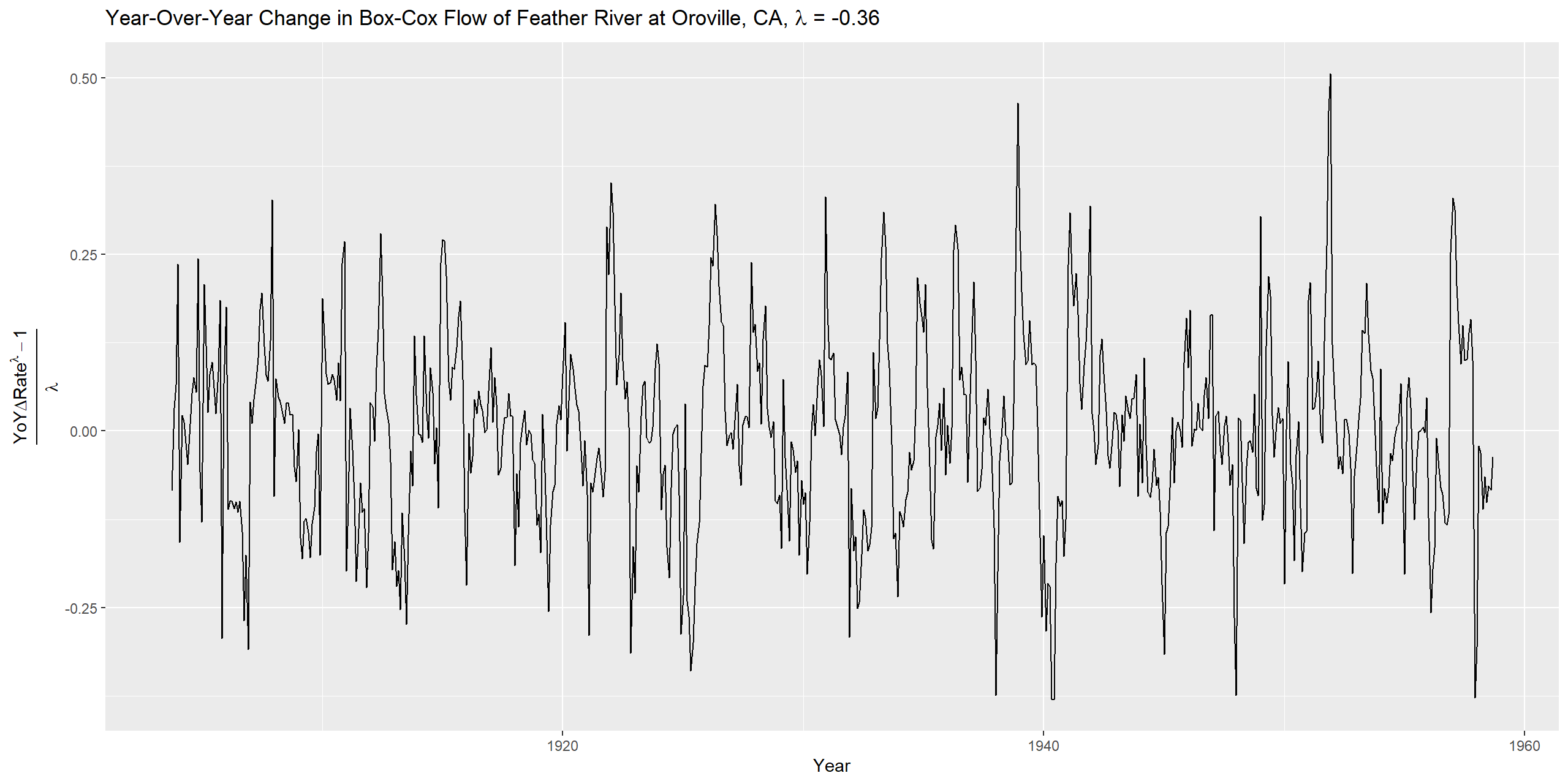<!DOCTYPE html>

STAT 4280 Final Project

## Data Set Title

## Feather River monthly flow rate in cubic meters/s at Oroville, California, Oct.1902 – Sep. 1961

The Feather River is the principal tributary of the Sacramento River, which discharges into the Suisun Bay, a tidal estuary of the San Francisco Bay Area. The measurements in the time series, according to the Time Series Data Library, were conducted between 10/1902 and 09/1977, but the data actually only appears to be complete through 09/1961, the same year which construction on the Oroville Dam began in earnest; the partially-completed Oroville Dam mitigated the local impact of the Pacific Northwest-wide Christmas floods of 1964, which caused a peak flow of 7100 $\frac{m^{3}}{s}$. The Oroville Dam is the tallest dam in the US, at 235 m in height, and uses 480 $\frac{m^{3}}{s}$ of its flow limit of 4200 $\frac{m^{3}}{s}$ to generate power.

## Time series of data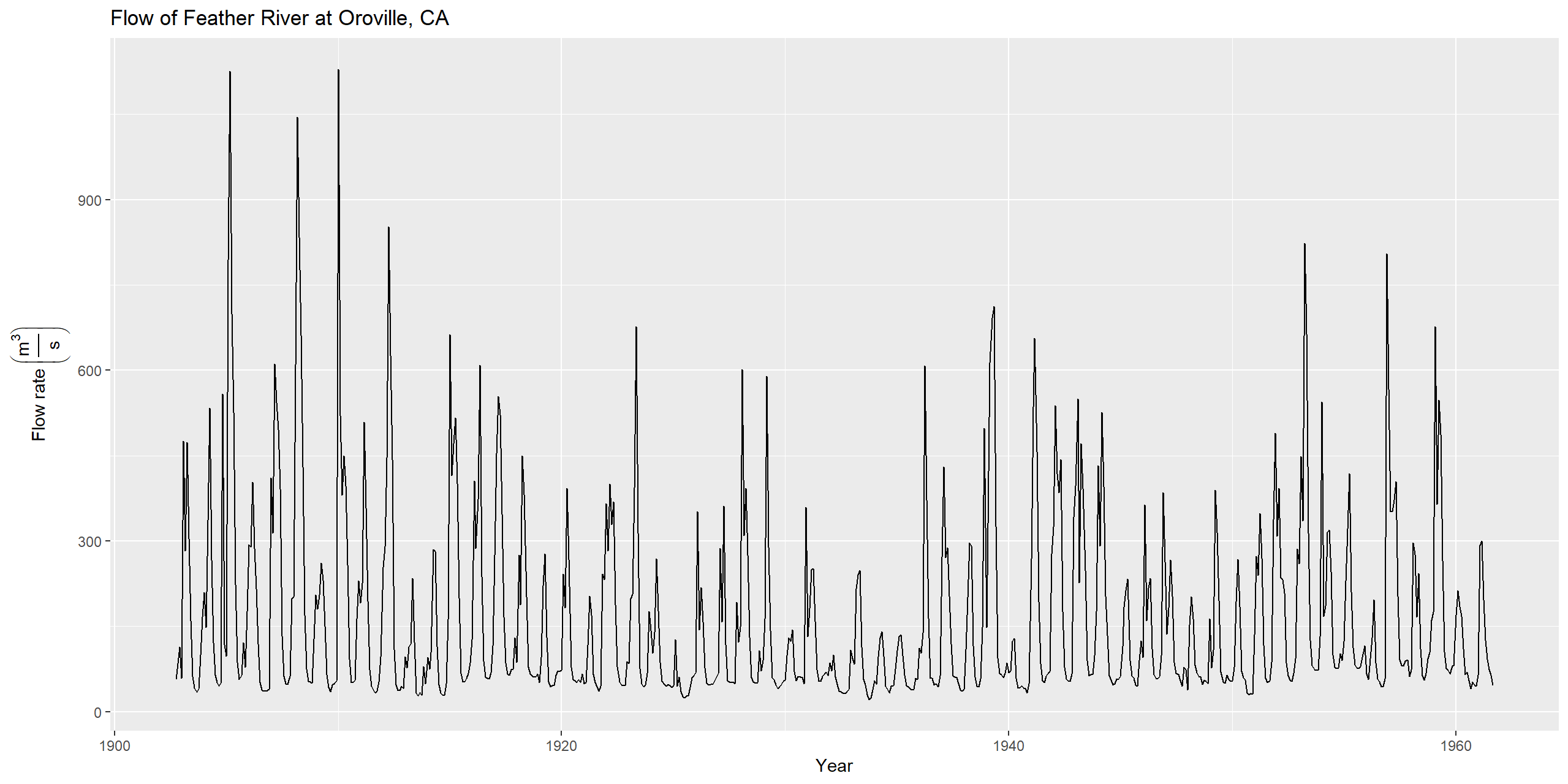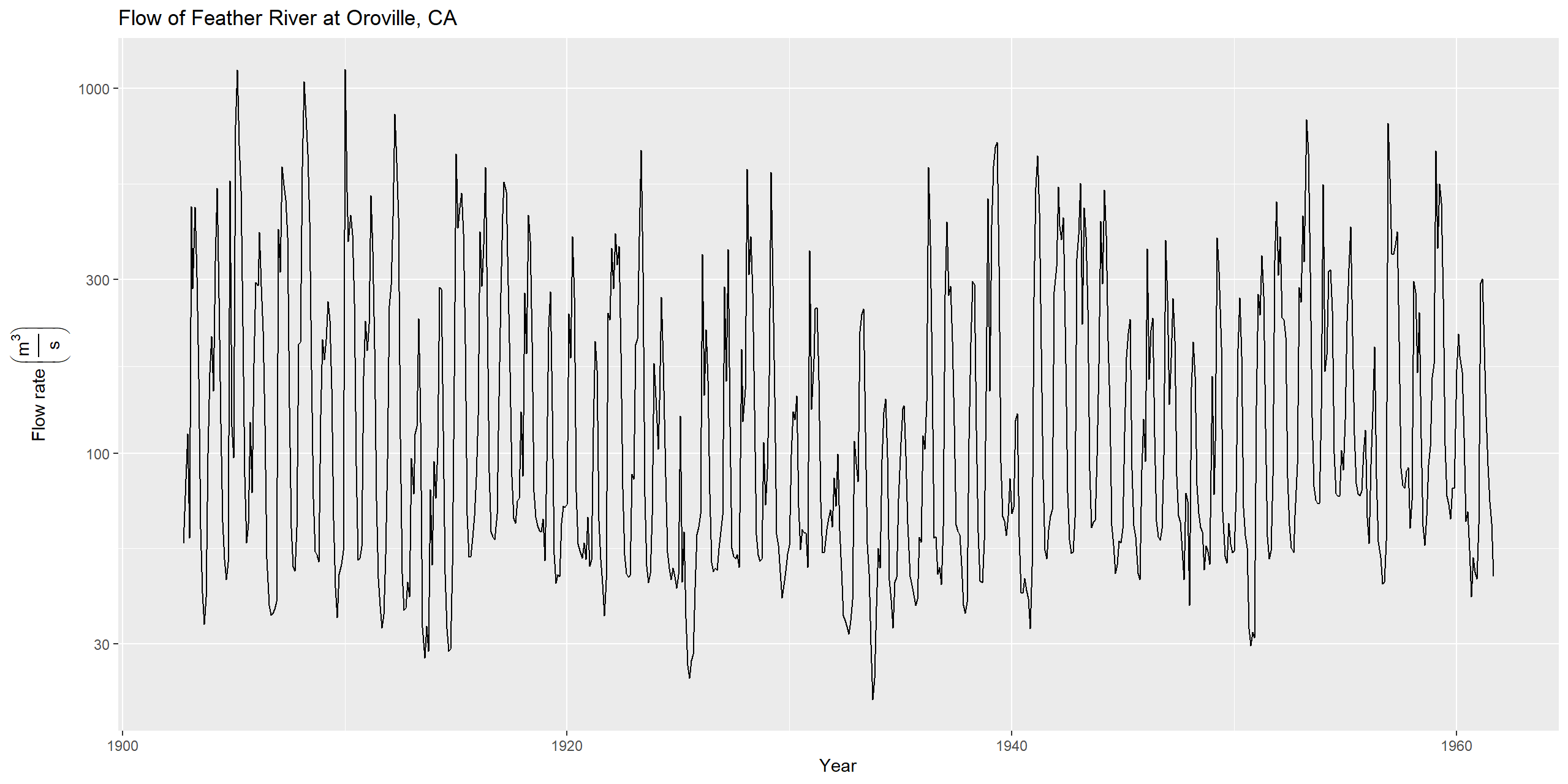The data need a variable transformation in order to stabilize the variance; a Box-Cox analysis should optimize the $\lambda$ necessary to stabilize the variance as much as possible.

## Box-Cox Transformation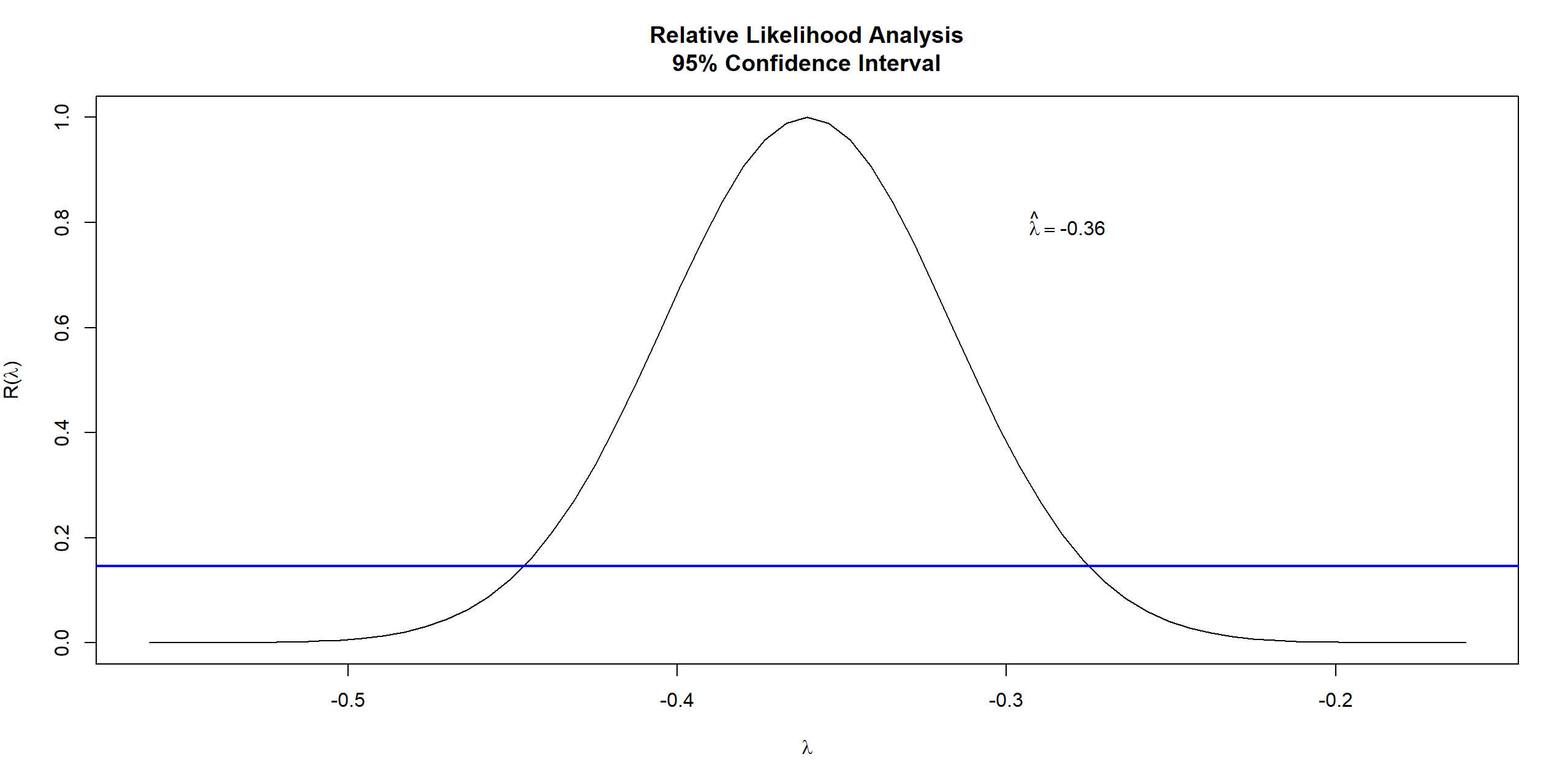## Analysis Techniques

The data is split into training (n=672) and testing (n=36) datasets for most graphical comparison of prediction errors, though different testing-training splits are necessary to evaluate the consistency of the quality of the models. SARIMA models are chosen using analysis of the periodogram and the sample’s year-over-year $(1-B^{12})$, and differenced year-over-year $(1-B)(1-B^{12})$ ACF and PACF graphs. Quality of the SARIMA models is evaluated by comparing AICs, BICs, RMSE, MAPE, and MRPE, the latter of which is calculated as follows:

$\frac{1}{t_{max}} \sum_{1}^{t_{max}}\frac{|Prediction_{i} - Actual_{i}|}{Actual_{i}}$

Once a final candidate SARIMA model is found, then the best models through LOWESS decomposition and exponential smoothing are found. The LOWESS models are compared by RMSE across different variable transformations (with RMSE being representative of the gap between actual and the model’s detransformed seasonal+trend parts), and the predictions are compared across the four types (ETS, ARIMA, naive (i.e. no evolution in predicted points), and random walk with drift), resulting in 12 possible models that may provide the best MAPE for a given testing length. The exponential smoothing methods are compared by RMSE and MAPE across variable transformations and by seasonality calculation method (i.e. additive or multiplicative). The best models found through triple exponential smoothing and decomposition using the classical method and locally-weighted least-squares are compared to the SARIMA model.

## Analyzing the transformed data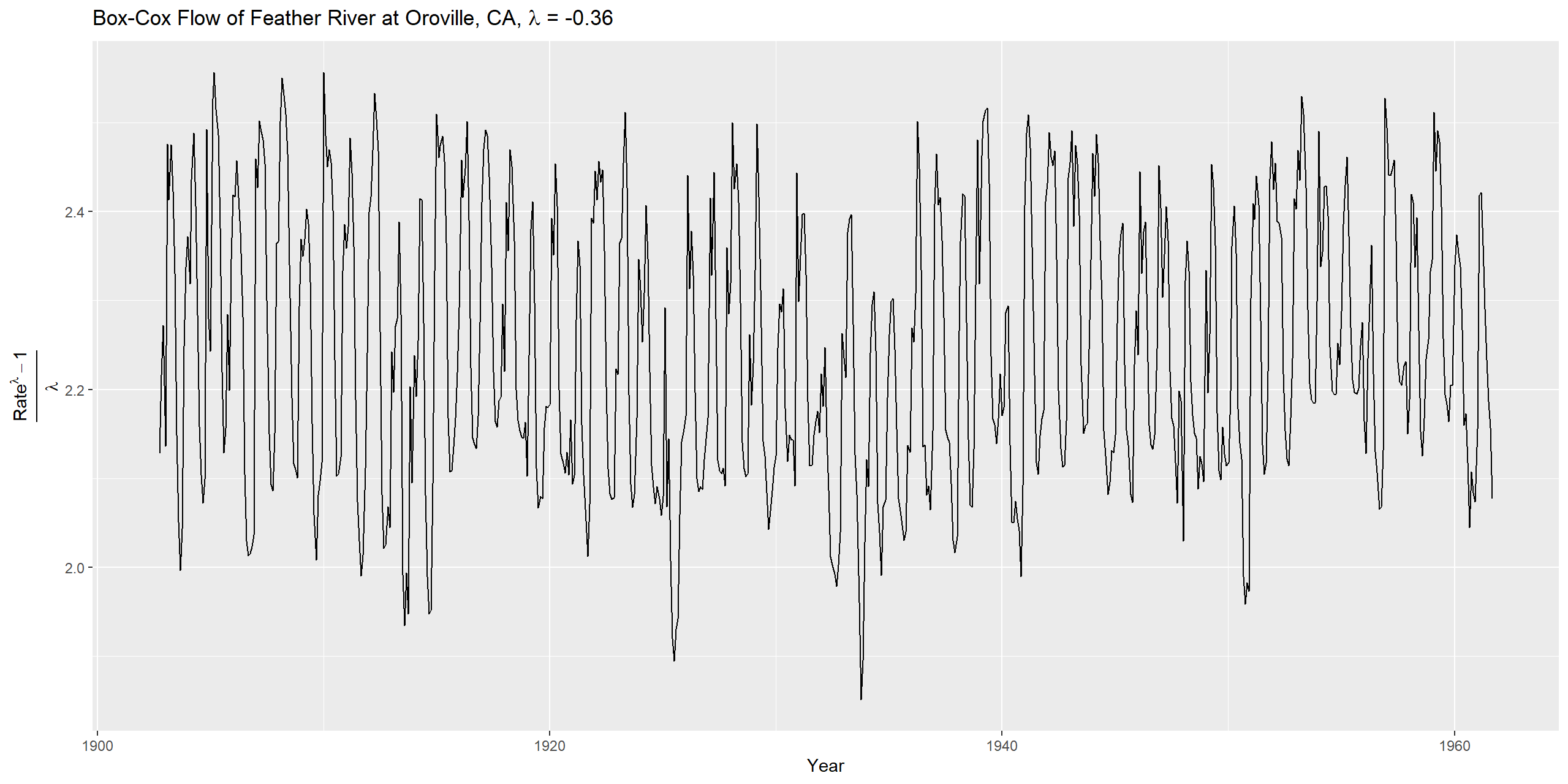The data appear to have a periodic nature, so analyzing the ACF, PACF, and periodogram are necessary to develop a candidate model.

## ACF and PACF of transformed data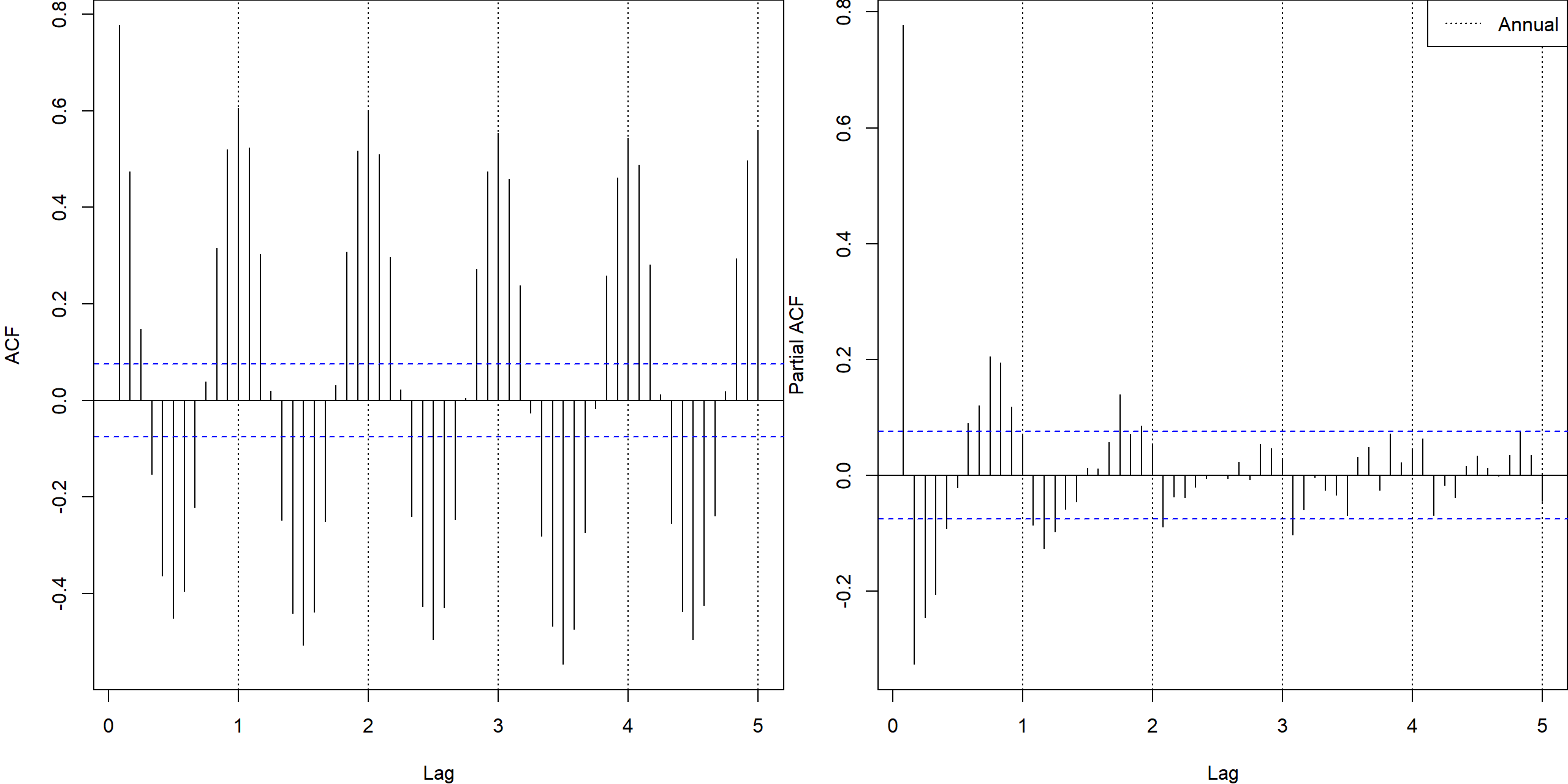## Analysis of ACF, PACF

The ACF has a persistent sinusoidal pattern, with peaks at lags with annual recurrence, troughs lagged six months behind, similar to a cosine wave with weak decay. The PACF has a dampened sinusoidal pattern, similar to $-e^{-kx}sin{\frac{\pi x}{6}}$ but because $\phi_{11}=\rho(X_{t},X_{t-1})$ the first partial autocorrelation is positive.

## Periodogram of Data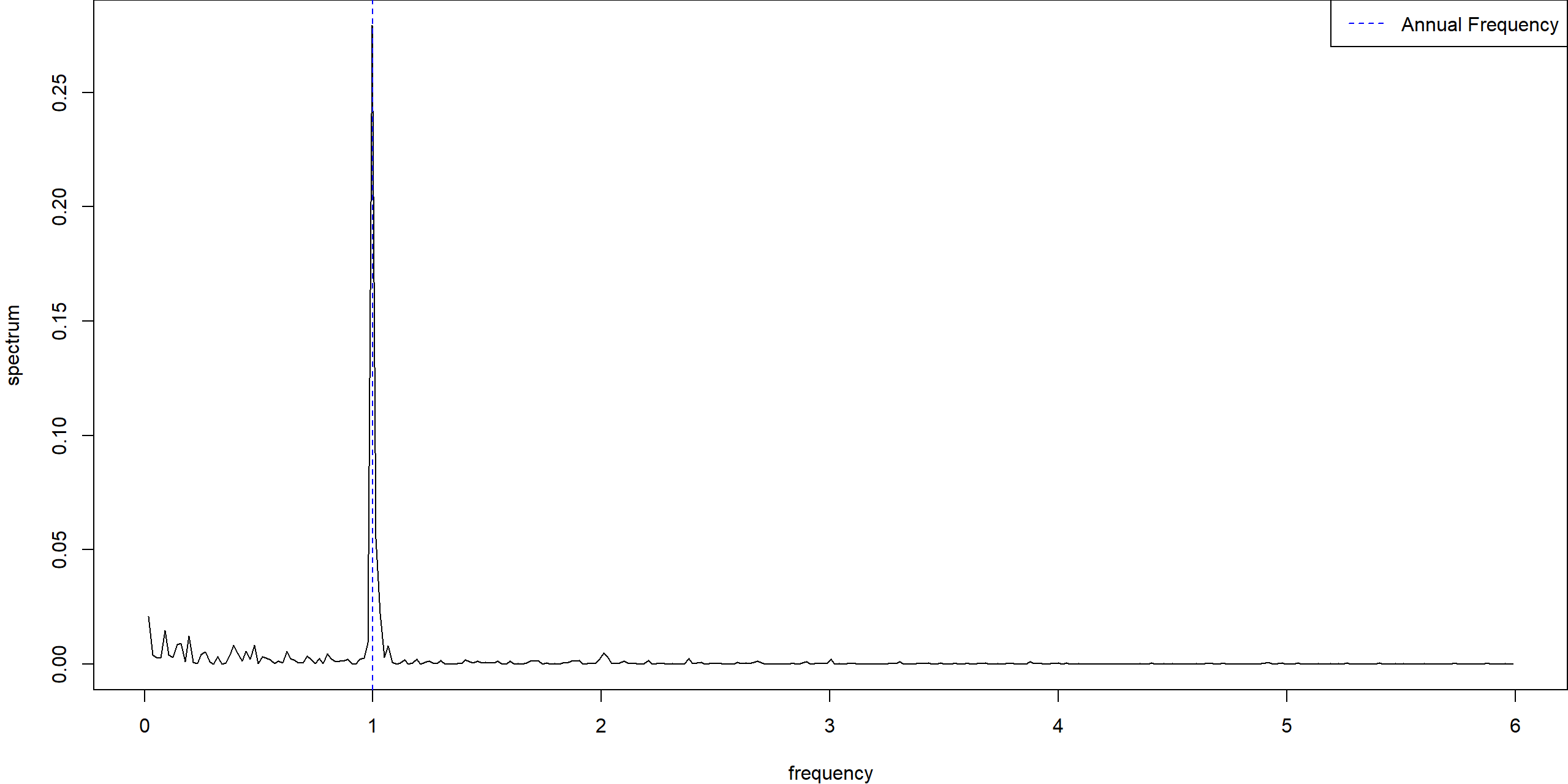The periodogram has a strong spike at annual recurrence, so investigating the annual differences is necessary.

## Year-over-year changes